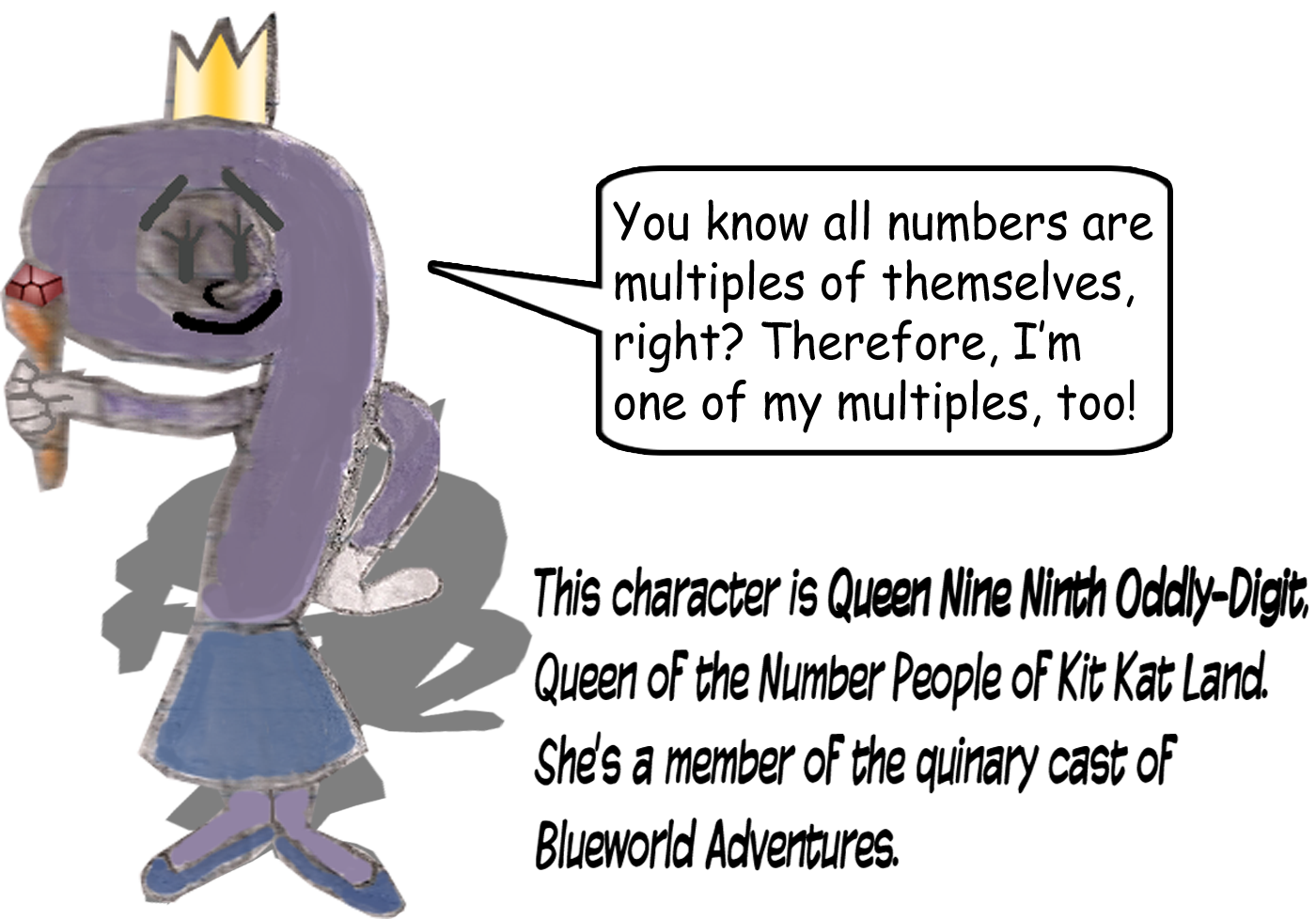# Nifty Number Nine & Its Multiples

## This trick isn't just about the number 9 & its multiples, but also about 2-digit numbers. 9 & its multiples have the power to swap the digits of 2-digit numbers! All it takes is some addition or subtraction.

12 + 9 = 21

21 - 9 = 12

### If the bigger number is to the left of 9x, then you should subtract; if the smaller number is to the left of 9x, then you should add.## This next example uses 18, which is 9 × 2.

35 + 18 = 53

53 - 18 = 35

### There's something you have to do with the digits of the 2-digit numbers to know which multiple of 9 you have to add or subtract to swap the ones digit & tens digit of the 2-digit number you picked: simply multiply the absolute value of the difference of the 2 digits by 9.

9(|T - D|) = M

T = tens digit, D = ones digit, M = the multiple of 9 that swaps the digits!

## 9 × 2 = 18

...And there's the multiple of 9 that had to be used in the previous example!

## But I'll show you a few more!

58 + 27 = 85

85 - 27 = 58

(|5 - 8| = |8 - 5| = 3; 9 × 3 = 27)

16 + 45 = 61

61 - 45 = 16

(|1 - 6| = |6 - 1| = 5; 9 × 5 = 45)

29 + 63 = 92

92 - 63 = 29

(|2 - 9| = |9 - 2| = 7; 9 × 7 = 63)

19 + 72 = 91

91 - 72 = 19

(|1 - 9| = |9 - 1| = 8; 9 × 8 = 72)

09 + 81 = 90

90 - 81 = 09

(|0 - 9| = |9 - 0| = 9; 9 × 9 = 9^2 = 81)